Home | | Electronic Circuits I | Diode and Thermistor Compensation technique

# Diode and Thermistor Compensation technique

It refers to the use of temperature sensitive devices such as diodes, transistors, thermistors which provide compensating voltage and current to maintain Q point stable.

Compensation technique:

It refers to the use of temperature sensitive devices such as diodes, transistors, thermistors which provide compensating voltage and current to maintain Q point stable.

1.     Diode Compensation Techniques

Compensation for VBE:

a) Diode in Emitter Circuit

Diagram shows the voltage divider bias with bias compensation technique.Here, separate supply VDD is used to keep diode in forward If biased condition. If the diode used in the circuit is of same material and type as the transistor, the voltage across the diode will have the same temperature coefficient as the base to emitter voltage VBE . So when VBE changes by ∂ VBE with change in temperature, VD changes by VD and ∂ VD~=~∂ VBE, the changes tend to cancel each other. Apply*g KVL to the base circuit of Fig. ,we haveFigure: Stabilization by means of voltage divider bias and diode Compensation TechniqueAs VD tracks VBE with respect to temperature it is clear that IC will be insensitive to variations in VBE .

Diode in voltage divider circuitDiode is connected in series with resistance R2 in the voltage divider circuit and it is forward biased condition.

For voltage divider bias,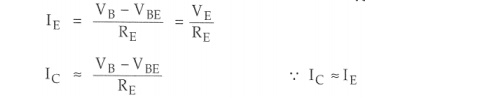When VBE changes with temperature, IC also changes

To cancel the changes in IC , one diode is used in the circuit for compensation

The voltage at the base VB is give asSubstituting this value in equation IC, we get,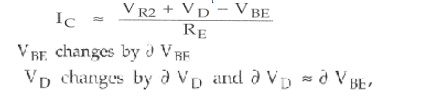The changes cancel each other , so the collector current is given as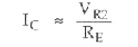The changes in VBE. Due to temperature are compensated by changes in the diode voltage which keeps IC stable at Q point.

Compensation for ICO

*         In germanium transistor changes in ICO with temperature plays an important role collector current stability

*        The diode is kept at reverse bias condition ,so only leakage current flows

*        Io  increases then ICO also increasesAs I is constant , IC also remains constant. We can say that changes by ICO with temperature are compensated by diode and collector current remains constant

2. Thermistor CompensationWith increase of temperature ,RT decreases. Hence the voltage drop across it also decreases. That is VBE decreases which reduces IB .this will offset the increased collector current with temperature.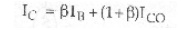The equation shows if there is increase in ICO and decrease in IB keeps IC almost constant.Fig (b) shows another thermistor compensation technique . Here, thermistor is connected between emitter and Vcc to minimize the increase in collector current due to changes in ICO, VBE, or beta with temperature .IC increases with temperature and RT decreases with increase in temperature. Therefore, current flowing through RE increases, which increases the voltage drop across it. E - B junction is forward biased. But due to increase in voltage drop across RE, emitter is made more positive, which reduces the forward bias voltage VBE. Hence, bias current reduces.As Ico increases with temperature, IB decreases and hence. IC remains constant

Sensistor Compensation techniqueThis method of transistor compensation uses temperature sensitive resistive element, sensistors rather than diodes or transistors. It has a positive temperature coefficient, its resistance increases exponentially with increasing temperature as shown in the Fig

Slope of this curve =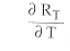is the temperature coefficient for thermistor and the slope is positive So we can say that sensistor has positive temperature coefficient of resistance (PTC).

Fig. shows sensistor compensation R1 is replaced by sensistor RT in self bias circuit. Now, RT and R2 resistors of the potential divider. As temperature increases, RT increases which decreases the current flowing through it. Hence current through R2 decreases which reduces the voltages drop across it. Voltage drop across R2 is the voltage between base and ground. So VBE reduces which decreases 16. It means, when ICBO increases with increase in temperature, IB reduces due to reduction in VBE, maintaining IC fairly constant.

Study Material, Lecturing Notes, Assignment, Reference, Wiki description explanation, brief detail
Electronic Circuits : Biasing of Discrete BJT and MOSFET : Diode and Thermistor Compensation technique |